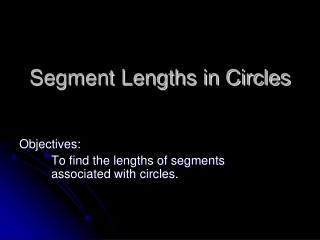DownloadDownload PresentationSegment Lengths in Circles

# Segment Lengths in Circles

Download Presentation## Segment Lengths in Circles

- - - - - - - - - - - - - - - - - - - - - - - - - - - E N D - - - - - - - - - - - - - - - - - - - - - - - - - - -
##### Presentation Transcript

1. Segment Lengths in Circles Objectives: To find the lengths of segments associated with circles.

2. Secants F B A E • Secant – A line that intersects a circle in exactly 2 points. • EF or AB are secants • AB is a chord

3. Lengths of Secants, Tangents, & Chords 2 Secants 2 Chords Tangent & Secant y a c t z x b z d w y a•b = c•d t2 = y(y + z) w(w + x) = y(y + z)

4. Find length of x. Find the length of g. Examples: 8 15 g 3 x 7 5 t2 = y(y + z) 152 = 8(8 + g) 225 = 64 + 8g 161 = 8g 20.125 = g a•b = c•d (3)•(7) = (x)•(5) 21 = 5x 4.2 = x

5. Ex.: 2 Secants Find the length of x. 20 14 16 w(w + x) = y(y + z) 14(14 + 20) = 16(16 + x) (34)(14) = 256 + 16x 476 = 256 + 16x 220 = 16x 3.75 = x x

6. Ex. : A little bit of everything! Find the measures of the missing variables Solve for k first. w(w + x) = y(y + z) 9(9 + 12) = 8(8 + k) 186 = 64 + 8k k = 15.6 12 k 175° 9 8 60° Next solve for r t2 = y(y + z) r2 = 8(8 + 15.6) r2 = 189 r = 13.7 a° r Lastly solve for ma m1 = ½(x - y) ma = ½(175 – 60) ma = 57.5°

7. What have we learned? • When dealing with angle measures formed by intersecting secants or tangents you either add or subtract the intercepted arcs depending on where the lines intersect. • There are 3 formulas to solve for segments lengths inside of circles, it depends on which segments you are dealing with: Secants, Chords, or Tangents.